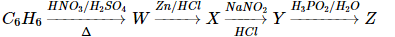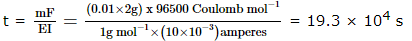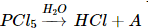JEE  >  WBJEE Chemistry Test - 12

# WBJEE Chemistry Test - 12

Test Description

## 40 Questions MCQ Test WBJEE Sample Papers, Section Wise & Full Mock Tests | WBJEE Chemistry Test - 12

WBJEE Chemistry Test - 12 for JEE 2022 is part of WBJEE Sample Papers, Section Wise & Full Mock Tests preparation. The WBJEE Chemistry Test - 12 questions and answers have been prepared according to the JEE exam syllabus.The WBJEE Chemistry Test - 12 MCQs are made for JEE 2022 Exam. Find important definitions, questions, notes, meanings, examples, exercises, MCQs and online tests for WBJEE Chemistry Test - 12 below.
Solutions of WBJEE Chemistry Test - 12 questions in English are available as part of our WBJEE Sample Papers, Section Wise & Full Mock Tests for JEE & WBJEE Chemistry Test - 12 solutions in Hindi for WBJEE Sample Papers, Section Wise & Full Mock Tests course. Download more important topics, notes, lectures and mock test series for JEE Exam by signing up for free. Attempt WBJEE Chemistry Test - 12 | 40 questions in 60 minutes | Mock test for JEE preparation | Free important questions MCQ to study WBJEE Sample Papers, Section Wise & Full Mock Tests for JEE Exam | Download free PDF with solutions
 1 Crore+ students have signed up on EduRev. Have you?
WBJEE Chemistry Test - 12 - Question 1

### NH₄Cl in liquid NH₃ will act as a/an :

WBJEE Chemistry Test - 12 - Question 2

### The compound 'A' when treated with methyl alcohol and few drops of H₂SO₄ give wintergreen smell. The compound 'A' is

WBJEE Chemistry Test - 12 - Question 3

### Scurvy is caused due to deficiency of

WBJEE Chemistry Test - 12 - Question 4
In Rosenmund reaction,
WBJEE Chemistry Test - 12 - Question 5
Which of the following is soluble in yellow ammonium sulphide?
WBJEE Chemistry Test - 12 - Question 6
The phenomenon in which white transparent crystal changes into white powder is called
WBJEE Chemistry Test - 12 - Question 7
What is the wave number of 4th line in Balmer series of Hydrogen spectrum? (R = 1,09,677cm-1)
WBJEE Chemistry Test - 12 - Question 8

Which of the following does not have sp2-hybridised carbon?

WBJEE Chemistry Test - 12 - Question 9
Which pair of elements among the following will form most stable ionic bond
WBJEE Chemistry Test - 12 - Question 10
Which among the following is not a state function ?
WBJEE Chemistry Test - 12 - Question 11
A vessel at equilibrium, contains SO₃, SO₂ and O₂. Now some helium gas is added, so that total pressure increases while temperature and volume remain constant. According to Le-Chatelier's principle, the dissociation of SO₃
WBJEE Chemistry Test - 12 - Question 12
A first order reaction is 10% complete in 20 min. The time taken for 19% completion is
WBJEE Chemistry Test - 12 - Question 13
The systematic name of (CH₃)₂CH-COOH is
WBJEE Chemistry Test - 12 - Question 14
Diazo coupling is useful to prepare some
WBJEE Chemistry Test - 12 - Question 15

Identify the reaction for which Δ H ≠ Δ E

WBJEE Chemistry Test - 12 - Question 16
Which among the following is the strongest base?
WBJEE Chemistry Test - 12 - Question 17

The chemical formula of Iron (III) hexacyanoferrate (II) is

WBJEE Chemistry Test - 12 - Question 18

0.24 g of a volatile gas upon vapourisation gives 45 ml vapour of N.T.P. what will be vapour density of the substance ? (Density of H₂ = 0.089 gl-1)

WBJEE Chemistry Test - 12 - Question 19

Which one of the following is correct about stability of the given ions?

WBJEE Chemistry Test - 12 - Question 20
When bauxite powder is mixed with coke and reacted with nitrogen at 2075K, carbon compound and X are formed. What is the gas formed when X is reacted with water
WBJEE Chemistry Test - 12 - Question 21
Which of the following substances is used as fertilizer ?
WBJEE Chemistry Test - 12 - Question 22
Reaction of potassium with water is
WBJEE Chemistry Test - 12 - Question 23
The number of π-bonds in CH₂ = CH-CH = CH-C ≡ CH is
WBJEE Chemistry Test - 12 - Question 24
The half-life of a radioactive isotope is 2.3 hours. Its disintegration constant will be
WBJEE Chemistry Test - 12 - Question 25
Which of the following is plasticizer
WBJEE Chemistry Test - 12 - Question 26
Aniline in a set of reactions yielded a product D

The structure of D is
WBJEE Chemistry Test - 12 - Question 27
Buna-N is prepared by the polymerisation of
WBJEE Chemistry Test - 12 - Question 28
In which of the following reaction there is no change in oxidation number
WBJEE Chemistry Test - 12 - Question 29

The second order Bragg diffraction of X-rays with λ = 1.00 Å from a set of parallel planes in a metal occurs at an angle of 600. The distance between the scattering planes in the crystal is

WBJEE Chemistry Test - 12 - Question 30
Consider the following statements about chirality :
1. Molecules which are not superimposable on their mirror images are achiral
2. A chiral molecule can have simple axis of symmetry
3. A carbon atom of which four different groups are attached is a chiral centre
4. A compound whose molecules are achiral exhibits optical activity
Which of the statements given above are correct ?
WBJEE Chemistry Test - 12 - Question 31

Solubility product constants (K(sp)) of salts of types MX, MX2 and M3X at temperature 'T' are 4.0 x 10-8, 3.2 x 10-14 and 2.7 x 10-15, respectively. Solubilities (mol dm-3) of the salts at temperature 'T' are in the order

WBJEE Chemistry Test - 12 - Question 32

'Z' in the following sequence of reactions isWBJEE Chemistry Test - 12 - Question 33

Electrolysis of dilute aqueous NaCl solution was carried out by passing 10 milli ampere current. The time required to liberate 0.01 mol of H2 gas at the cathode is (1 Faraday = 96500 C mol-1)

Detailed Solution for WBJEE Chemistry Test - 12 - Question 33

According to Faraday's first law of electrolysis:
m = EIt/F
Where:
m = mass of substance
E = equivalent weight of substance
I = current in amperes
t = time required
F = 96,500 Coulombs
t = mF EI = (0.01x 2g) x 96500 CoulombWBJEE Chemistry Test - 12 - Question 34

In the following reactionthe product 'A' is

WBJEE Chemistry Test - 12 - Question 35

Both [Ni(CO)4] and [Ni(CN)4](2-) are diamagnetic. The hybridisations of nickel in these complexes, respectively, are

WBJEE Chemistry Test - 12 - Question 36

Optical activity is expected for :

WBJEE Chemistry Test - 12 - Question 37

Chlorine atom does not differ from chloride ion in the number of which of the following?

WBJEE Chemistry Test - 12 - Question 38

Which of the following statements is/are true about an azeotropic mixture?

WBJEE Chemistry Test - 12 - Question 39

If x and y are arbitrary extensive variables, then

WBJEE Chemistry Test - 12 - Question 40

If Pand Ps are the V.P. of solvent and its solution respectively and N1 and N2 are the moles of solute and solvent then

## WBJEE Sample Papers, Section Wise & Full Mock Tests

4 videos|10 docs|54 tests
 Use Code STAYHOME200 and get INR 200 additional OFF Use Coupon Code
Information about WBJEE Chemistry Test - 12 Page
In this test you can find the Exam questions for WBJEE Chemistry Test - 12 solved & explained in the simplest way possible. Besides giving Questions and answers for WBJEE Chemistry Test - 12, EduRev gives you an ample number of Online tests for practice

## WBJEE Sample Papers, Section Wise & Full Mock Tests

4 videos|10 docs|54 tests